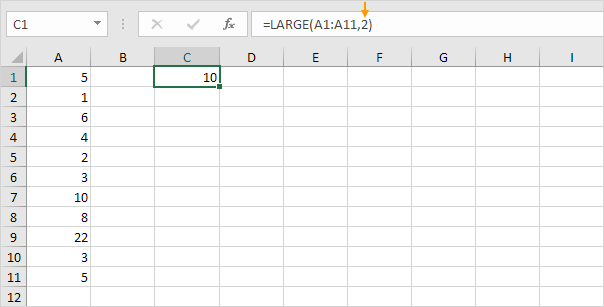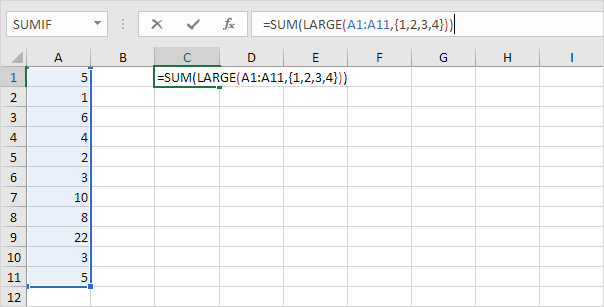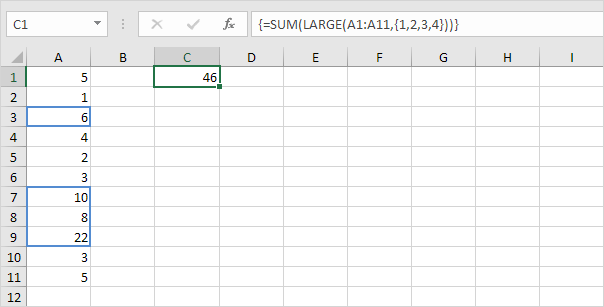# Sum Largest Numbers

This example shows you how to create an array formula that sums the largest numbers in a range.

1. We use the LARGE function. For example, to find the second largest number, use the following function.2. To sum the 4 largest numbers (don't be overwhelmed), add the SUM function and replace 2 with {1,2,3,4}.3. Finish by pressing CTRL + SHIFT + ENTER.Note: the formula bar indicates that this is an array formula by enclosing it in curly braces {}. Do not type these yourself. They will disappear when you edit the formula. Using Excel 365 or Excel 2021? Finish by simply pressing Enter. You won't see curly braces.

Explanation: the range (array constant) created by the LARGE function is stored in Excel's memory, not in a range. The array constant looks as follows.

{22,10,8,6}

This array constant is used as an argument for the SUM function, giving a result of 46.

Go to Next Chapter: Sort